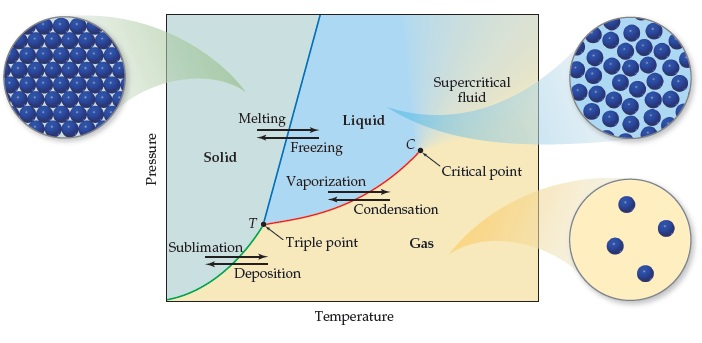# Problem: Generic phase diagram for a pure substance. The green line is the sublimation curve, the blue line is the melting curve, and the red line is the vapor-pressure curve.Imagine that the pressure on the solid phase in the figure is decreased at constant temperature. If the solid eventually sublimes, what must be true about the temperature?

###### FREE Expert Solution

We are asked what would be true about temperature if the pressure is decreased and the solid eventually sublimes.

Sublimation is the transition of a substance directly from the solid to the gas phase, without passing through the intermediate liquid phase.###### Problem DetailsGeneric phase diagram for a pure substance. The green line is the sublimation curve, the blue line is the melting curve, and the red line is the vapor-pressure curve.

Imagine that the pressure on the solid phase in the figure is decreased at constant temperature. If the solid eventually sublimes, what must be true about the temperature?